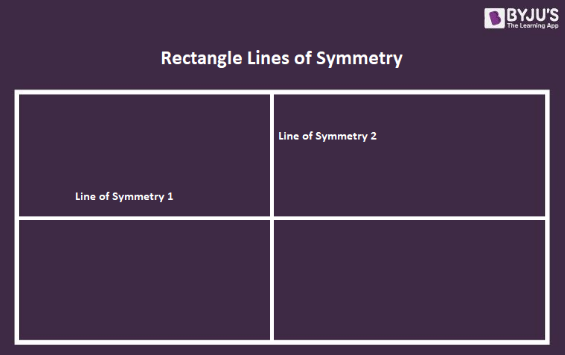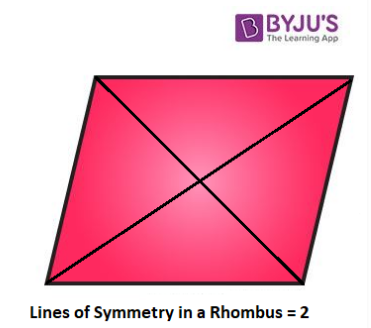# Lines Of Symmetry in a Parallelogram

Lines of symmetry in a parallelogram vary from type to type. In simple words, the parallelogram lines of symmetry refer to the lines which cut the parallelogram into two identical parts. To recall, a parallelogram is a quadrilateral (4-sided figure) where the opposite sides are parallel to each other.

## What are the Lines of Symmetry in Parallelograms?

The lines of symmetry are those lines which divide a parallelogram into two halves where each half is the mirror image of the other. Different parallelograms have different lines of symmetry and the different number of symmetry lines.

Symmetry Lines in Parallelograms
Parallelogram Name Number of Symmetry Lines
Square 4
Rectangle 2
Rhombus 2

There are three types of a parallelogram whose number of symmetry lines are given in the aforementioned table. Below are the explanations on the lines of symmetry in each of these parallelograms.

### Lines of Symmetry in a Square

In a square, there are four lines of symmetry, each of which divides it into two identical parts. The symmetry lines of a square are both its diagonals and the lines joining the midpoints of its opposite sides (bisectors).### Lines of Symmetry in a Rectangle

There are two lines of symmetry in a rectangle which cuts it into two equal halves. In a rectangle, the lines of symmetry are those lines which join the midpoint of the opposite and parallel lines (i.e. the bisector) of the rectangle.### Lines of Symmetry in a Rhombus

In a rhombus, the lines of symmetry are its diagonals. So, the number of symmetry lines in a rhombus are two i.e. its diagonals which divide it into two identical halves where each part is the mirror image of the other.It should be noted that a figure shows symmetry only when the line of symmetry divides the figure in a way that both the halves become the mirror image of each other. So, in a rectangle and a rhombus, it is seen that the lines of symmetry are not the same as that of the square.

In any figure, there can be multiple lines of symmetry. Thus, it is important to check whether the lines of symmetry divide the figure not only in equal parts but as mirror images also.

## Rotational Symmetry in Parallelograms

Rotational symmetry is the symmetry wherein the figure retains its exact appearance after it is rotated around a centre point. Different parallelograms have different orders of rotational symmetry which are mentioned below.

Order of Rotational Symmetry in Parallelograms
Parallelogram Name Order of Rotational Symmetry Angle of Rotation
Square 4 90°, 180°, 270° and 360°
Rectangle 2 180° and 360°
Rhombus 2 180° and 360°

## Frequently Asked Questions on Lines Of Symmetry in a Parallelogram

### What is a Parallelogram?

A parallelogram is a quadrilateral whose opposite sides are equal and parallel. There are 3 main types of parallelograms which are square, rectangle and rhombus.

### How Many Lines of Symmetry are There in Parallelograms?

Different parallelograms have different numbers of symmetry lines.

Square = 4

Rectangle = 2

Rhombus = 2

### Which Parallelogram has the Most Number of Symmetry Lines?

A square has the most number of symmetry lines which is equal to 4.

What are the Symmetry Lines in Different Parallelograms?

The symmetry lines of the parallelograms are:

Square = diagonals, bisectors of opposite sides

Rectangle = bisectors of opposite sides

Rhombus = diagonals

Keep visiting BYJU’S to learn more such maths topics in an easy and engaging way. Also, download BYJU’S- The Learning App to get a personalised and effective learning experience.

Quiz on Lines of symmetry in a parallelogram